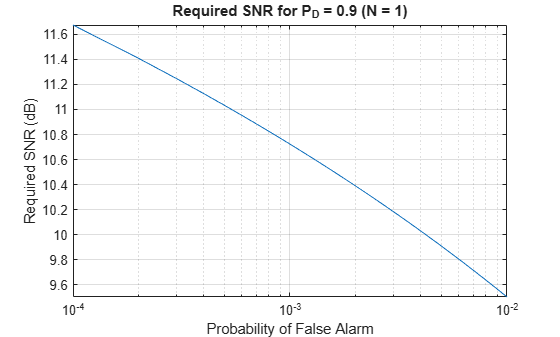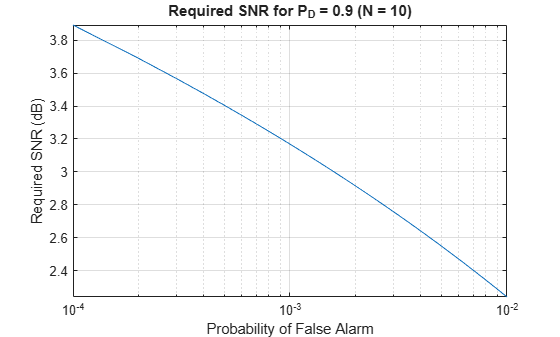Main Content

# albersheim

Required SNR using Albersheim’s equation

## Syntax

```SNR = albersheim(prob_Detection,prob_FalseAlarm) SNR = albersheim(prob_Detection,prob_FalseAlarm,N) ```

## Description

`SNR = albersheim(prob_Detection,prob_FalseAlarm)` returns the signal-to-noise ratio in decibels. This value indicates the ratio required to achieve the given probabilities of detection `prob_Detection` and false alarm `prob_FalseAlarm` for a single sample.

`SNR = albersheim(prob_Detection,prob_FalseAlarm,N)` determines the required SNR for the noncoherent integration of `N` samples.

## Examples

collapse all

Compute the required SNR of a single pulse to achieve a detection probability of 0.9 as a function of the false alarm probability.

Set the probability of detection to 0.9 and the probabilities of false alarm from .0001 to .01.

```Pd=0.9; Pfa=0.0001:0.0001:.01;```

Loop the Albersheim equation over all Pfa's.

```snr = zeros(1,length(Pfa)); for j=1:length(Pfa) snr(j) = albersheim(Pd,Pfa(j)); end```

Plot SNR versus Pfa.

```semilogx(Pfa,snr,'k','linewidth',1) grid axis tight xlabel('Probability of False Alarm') ylabel('Required SNR (dB)') title('Required SNR for P_D = 0.9 (N = 1)')```Compute the required SNR of 10 non-coherently integrated pulse to achieve a detection probability of 0.9 as a function of the false alarm probability.

Set the probability of detection to 0.9 and the probabilities of false alarm from .0001 to .01.

```Pd=0.9; Pfa=0.0001:0.0001:.01; Npulses = 10;```

Loop over the Albersheim equation over all Pfa's.

```snr = zeros(1,length(Pfa)); for j=1:length(Pfa) snr(j) = albersheim(Pd,Pfa(j),Npulses); end```

Plot SNR versus Pfa.

```semilogx(Pfa,snr,'k','linewidth',1) grid axis tight xlabel('Probability of False Alarm') ylabel('Required SNR (dB)') title('Required SNR for P_D = 0.9 (N = 10)')```## More About

collapse all

### Albersheim's Equation

Albersheim's equation uses a closed-form approximation to calculate the SNR. This SNR value is required to achieve the specified detection and false-alarm probabilities for a nonfluctuating target in independent and identically distributed Gaussian noise. The approximation is valid for a linear detector and is extensible to the noncoherent integration of N samples.

Let

`$A=\mathrm{ln}\frac{0.62}{{P}_{FA}}$`

and

`$B=\mathrm{ln}\frac{{P}_{D}}{1-{P}_{D}}$`

where ${P}_{FA}$ and ${P}_{D}$ are the false-alarm and detection probabilities.

Albersheim's equation for the required SNR in decibels is:

`$\text{SNR}=-5{\mathrm{log}}_{10}N+\left[6.2+4.54/\sqrt{N+0.44}\right]{\mathrm{log}}_{10}\left(A+0.12AB+1.7B\right)$`

where `N` is the number of noncoherently integrated samples.

## References

 Richards, M. A. Fundamentals of Radar Signal Processing. New York: McGraw-Hill, 2005, p. 329.

 Skolnik, M. Introduction to Radar Systems, 3rd Ed. New York: McGraw-Hill, 2001, p. 49.

## See Also

Introduced in R2011a

## Support

#### Exploring Hybrid Beamforming Architectures for 5G Systems

Download white paper